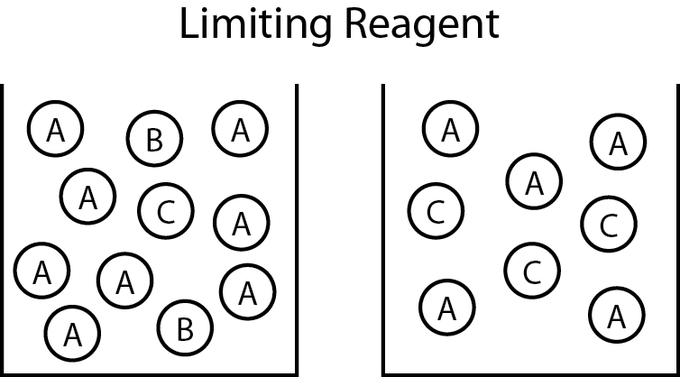## Limiting Reagents

#### Learning Objective

• Determine the limiting reagent and the amount of a product formed in a given reavion

#### Key Points

• The limiting reagent is the reactant that is used up completely. This stops the reaction and no further products are made.
• Given the balanced chemical equation that describes the reaction, there are several ways to identify the limiting reagent.
• One way to determine the limiting reagent is to compare the mole ratios of the amounts of reactants used. This method is most useful when there are only two reactants.
• The limiting reagent can also be derived by comparing the amount of products that can be formed from each reactant.

#### Term

• limiting reagentThe reactant in a chemical reaction that is consumed first; prevents any further reaction from occurring.

In a chemical reaction, the limiting reagent, or limiting reactant, is the substance that has been completely consumed when the chemical reaction is complete. The amount of product produced by the reaction is limited by this reactant because the reaction cannot proceed further without it; often, other reagents are present in excess of the quantities required to to react with the limiting reagent. From stoichiometry, the exact amount of reactant needed to react with another element can be calculated. However, if the reagents are not mixed or present in these correct stoichiometric proportions, the limiting reagent will be entirely consumed and the reaction will not go to stoichiometric completion.Limiting reagentThe limiting reagent in a reaction is the first to be completely used up and prevents any further reaction from occurring. In this reaction, reactant B is the limiting reagent because there is still some left over A in the products. Therefore, A was in excess when B was all used up.

## Determining the Limiting Reagent

One way to determine the limiting reagent is to compare the mole ratio of the amount of reactants used. This method is most useful when there are only two reactants. One reactant (A) is chosen, and the balanced chemical equation is used to determine the amount of the other reactant (B) necessary to react with A. If the amount of B actually present exceeds the amount required, then B is in excess, and A is the limiting reagent. If the amount of B present is less than is required, then B is the limiting reagent.

To begin, the chemical equation must first be balanced. The law of conservation states that the quantity of each element does not change over the course of a chemical reaction. Therefore, the chemical equation is balanced when the amount of each element is the same on both the left and right sides of the equation. Next, convert all given information (typically masses) into moles, and compare the mole ratios of the given information to those in the chemical equation.

For example: What would be the limiting reagent if 75 grams of C2H3Br3 reacted with 50.0 grams of O2 in the following reaction:

$4 \ C_2H_3Br_3 + 11 \ O_2 \rightarrow 8 \ CO_2 + 6 \ H_2O + 6 \ Br_2$

First, convert the values to moles:

$75 \ g \times \frac{1 \ mole}{266.72 \ g} = 0.28 \ mol \ C_2H_3Br_3$

$50.0 \ g \times \frac{1 \ mol}{32 \ g} = 1.56 \ mol \ O_2$

It is then possible to calculate how much C2H3Br3 would be required if all the O2 is used up:

$1.56 \ mol \ O_2 \times \frac{4 \ mol \ C_2H_3Br_3}{11 \ mol \ O_2} = 0.567 \ mol \ C_2H_3Br_3$

This demonstrates that 0.567 mol C2H3Br3 is required to react with all the oxygen. Since there is only 0.28 mol C2H3Br3 present, C2H3Br3 is the limiting reagent.

Another method of determining the limiting reagent involves the comparison of product amounts that can be formed from each reactant. This method can be extended to any number of reactants more easily than the previous method. Again, begin by balancing the chemical equation and by converting all the given information into moles. Then use stoichiometry to calculate the mass of the product that could be produced for each individual reactant. The reactant that produces the least amount of product is the limiting reagent.

For example: What would be the limiting reagent if 80.0 grams of Na2O2 reacted with 30.0 grams of H2O in the reaction?

$2 \ Na_2O_2 + 2 \ H_2O \rightarrow 4 \ NaOH + O_2$

The comparison can be done with either product; for this example, NaOH will be the product compared. To determine how much NaOH is produced by each reagent, use the stoichiometric ratio given in the chemical equation as a conversion factor:

$\frac{4 \ mol \ NaOH}{2 \ mol \ Na_2O_2}$ and $\frac {4 \ mol \ NaOH}{2 \ mol \ H_2O}$

Then convert the grams of each reactant into moles of NaOH to see how much NaOH each could produce if the other reactant was in excess.

$80.0 \ g \ Na_2O_2 \times \frac {1 \ mol \ Na_2O_2}{77.98 \ g \ Na_2O_2} \times \frac {4 \ moles \ NaOH}{2 \ mol \ Na_2O_2} = 2.06 \ moles \ NaOH$

$30.0 \ g \ H_2O \times \frac {1 \ mol \ H_2O}{18 \ g \ H_2O} \times \frac {4 \ moles \ NaOH}{2 \ moles \ H_2O} = 3.33 \ moles \ NaOH$

Obviously the Na2O2 produces less NaOH than H2O; therefore, Na2O2 is the limiting reagent.